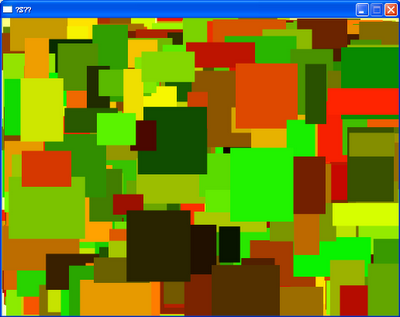## 2009年4月26日 星期日

### 色塊背景物件SetDim及GetDim是用來存取物件的範圍屬性，目前只使用到寬高二個值來決定色塊的大小。SetBgColor及GetBgColor則是用來存取色塊顏色值。

`math.randomseed(os.clock())for i = 1,2000 do  local cb = Good.GenObj(-1, 0, 'TestBlock')end`

TestBlock定義如下。
`TestBlock = {}TestBlock.OnCreate = function(param)  if (math.random(2) == 1) then    param.dirx = -1 * math.random(1,5)  else    param.dirx = 1 * math.random(1,5)  end  if (math.random(2) == 1) then    param.diry = -1 * math.random(1,5)  else    param.diry = 1 * math.random(1,5)  end  local id = param._id;  Good.SetBgColor(id, math.random(65535))  Good.SetDim(id, 0, 0, math.random(32,128), math.random(32,128))  Good.SetPos(id, math.random(0,600), math.random(0,440))endTestBlock.OnStep = function(param)  local id = param._id;  local x,y = Good.GetPos(id)  x = x + param.dirx  y = y + param.diry  Good.SetPos(id, x, y)  if (600 <= x or 0 >= x) then    param.dirx = -1 * param.dirx  end  if (440 <= y or 0 >= y) then    param.diry = -1 * param.diry  endend`